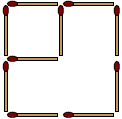This is a motivating speaking activity for lower levels to develop fluency.Author:
Katherine Bilsborough

In this activity, students work together to solve a 'cocktail stick' puzzle. Students are given plenty of support and use 'thinking time' before the speaking task. The lesson is usually successful with adults and teenagers because of the puzzle element. You will need a box of cocktail sticks.

Level

A1-B1

Age

Preparation

Take a box of cocktail sticks into the classroom. Arrange students around a central space with a table.

Procedure

Introduction

1. Use the cocktail sticks to make simple geometrical shapes and symbols. Point to each shape and ask What's this? Elicit an answer orally each time. Suggested shapes: a square, a triangle, a rectangle, a cross, a star, a diamond, a hexagon, etc.
2. Appoint a student to be your helper. Tell students that you are going to explain how to make a star shape with the cocktail sticks. Each time students hear a verb they should repeat it and the helper should write it on the board. Leave this list on the board for the students to refer to later on.
3. Make a star shape with the cocktail sticks, giving simple instructions as you make each move. Use as many different verbs as possible. Use affirmative and negative examples, e.g. Take a cocktail stick, place it here, put it there, pick up this cocktail stick, add another cocktail stick, don't move the other cocktail sticks, join these two sides, take away these two sticks, etc.

Model the activity

• Tell students they are going to do a puzzle. Make this shape using 12 cocktail sticks.• Write this puzzle on the board: Remove two cocktail sticks so that there are only two squares left.
• Tell students to think carefully about how to solve the puzzle and about the language they need to explain the solution. Elicit suggestions from the students. They should point at the sticks and give you clear instructions. Move the sticks as the students suggest until you have the answer.Pairwork

Students work in pairs, trying to solve another puzzle together, and then each pair of students works with another pair, to explain and check their ideas.

• Put students into pairs to work together. Give half the pairs handout A, and the other half handout B. Have a group of three if necessary to make an equal number of pairs.
• The pairs follow the instructions on their handouts. First they try to solve their own puzzle together, making suggestions. Then they discuss and make notes about the language they need to use to explain the solution to the other puzzle.
• Then Student A pairs get together with Student B pairs. Students A explain which moves they think need to be made to solve their puzzle. Students B (who have the solution) listen and tell them whether they are correct. If they are not, Students B can explain the answer using the notes they made. Then pairs change roles and Students B explain how they think their puzzle can be solved.Thinking time - a puzzle: handout AThinking time - a puzzle: handout B